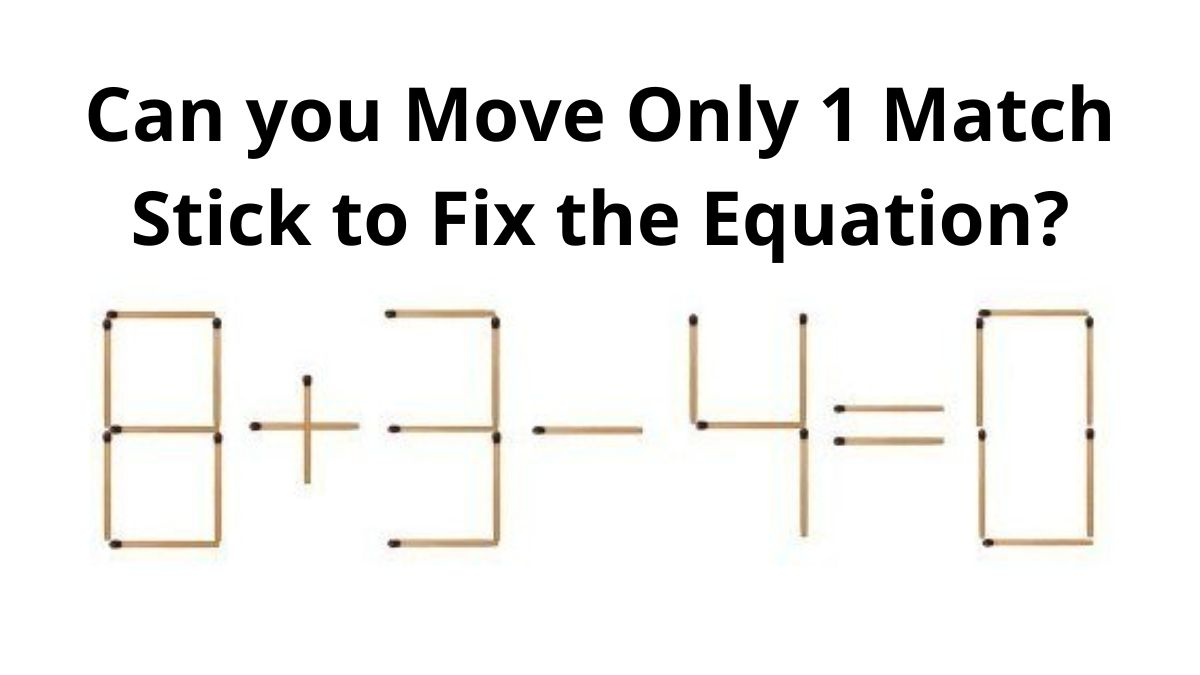# Brain Teaser Puzzle: Can you move only 1 Matchstick to fix the equation within 20 secs?

Brain Teaser Puzzle: In this Quiz, try to correct the equation by moving only 1 matchstick in the picture. Can you solve this riddle in 20 Seconds?Can you move only 1 Matchstick to fix the equation?

Can you move only 1 Matchstick to fix the equation? This brain teaser is for those who enjoy solving analytical puzzles and games. Brain teasers are mainly a trickier version of riddles and puzzles, as these brain games are solved with lateral thinking. While solving these, you need to analyze a little differently and out of the box. For coming on to the solution, you need to use a creative mind as the answer won’t be right in front of you. So, we have come up with an interesting brain-teaser where you have to move only 1 Matchstick to correct the equation in the image.

## Can you move only 1 Matchstick to fix the equation within 20 Seconds?

In the above image, you need to move one stick such that the equation becomes correct. An alert mind can solve this puzzle within 20 seconds. You need to analyze the equation and mathematical symbols carefully shown in the image. As a heads up, the answers to this brain teaser have been given right below the question, so make sure you don’t scroll too far and cheat!

Hint: Apply the rule: LHS = RHS.

In this brain puzzle, moving 1 matchstick from the left-hand side to the right-hand side will help you in coming up with an answer.

Given equation, 8 + 3 - 4 = 0 written with matchsticks

Pick a stick from the first number 8 such that it transforms to form number 9 and put that in the number of RHS such that it becomes 8.

By doing this we get,

9  + 3 - 4 = 8

So, the answer to the riddle is:

LHS = 9 + 3 - 4 = 12 - 4 = 8

RHS = 8

LHS = RHS

Check Most Popular Online Games 2022

Another answers to this Brain teaser can be:

Pick a stick from the first number 8 such that it transforms to form number 6 and put that in the number 4 such that it becomes 9.

LHS = 6+3-9=9-9=0

RHS = 0

LHS = RHS

Pick a stick from the third number 4 such that it transforms to form number 11.

LHS = 8+3-11=11-11=0

RHS = 0

LHS = RHS

Using lateral thinking will help you to derive answers in such brain teasers. This riddle was tricky but simple as it needs less time and brain power to solve. But it sure feels great when you figure out the answer in a few seconds.

Try 11 Wordle Alternative & Spin-Off Games Online

रोमांचक गेम्स खेलें और जीतें एक लाख रुपए तक कैश
Get the latest General Knowledge and Current Affairs from all over India and world for all competitive exams.